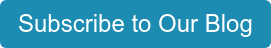There are many pitfalls of using Simple Payback Period (SPP) as a measure of a project’s merits. Ask yourself these seven questions:1.  Am I using the right figures?

- How did I calculate my costs?

- How did I calculate my savings?

2. What about the time value of money?

3. What about the period after the payback?

4. Must the project’s SPP be less than or equal to the holding period for the property into which the measures are being installed?

5. Can SPP reflect cash flow probabilities?

6. Do SPP “rules of thumb” even make sense?

7. In Landlord/Tenant settings, whose SPP am I calculating?

Just in case you are thinking, “Wait, but I use Return on Investment!” Return on Investment (ROI) is the reciprocal of SPP and suffers from very similar shortcomings. SPP is the first cost divided by Year 1 savings. ROI is Year 1 savings divided by first cost.

EXAMPLE:

\$100,000 investment saves \$25,000 in YR1
SPP = \$100,000 divided by \$25,000 = 4 years
ROI = \$25,000 divided by \$100,000 = 25%Read more blogs on Communication, Questioning, Prospecting

Posted by Mark Jewell